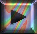# Stereochemistry

## Constitutional (structural) isomers

### Exercise 1Use skeletal formulas to represent all constitutional (structural) isomers a) with molecular formula $C_6H_{14}$. Does branching a hydrocarbon chain change the number of H atoms ?

c) with molecular formula $C_4H_6$. How the introduction of a triple bond in a hydrocarbon chain does affect the number of H atoms?

d) with molecular formula $C_4H_9Cl$. How the introduction of halogen atom does affect the number of H atoms of a hydrocarbon chain?

e) with molecular formula $C_3H_8O$. How the introduction of an oxygen atom by an alcohol or ether function does affect the number of H atoms of a hydrocarbon chain?

f) with molecular formula $C_3H_6O$. How the introduction of an oxygen atom by a carbonyl group does affect the number of H atoms?

→   All stereochemistry exercises